Learn all Concepts of Chapter 3 Class 10 (with VIDEOS). Check - Linear Equations in 2 Variables - Class 10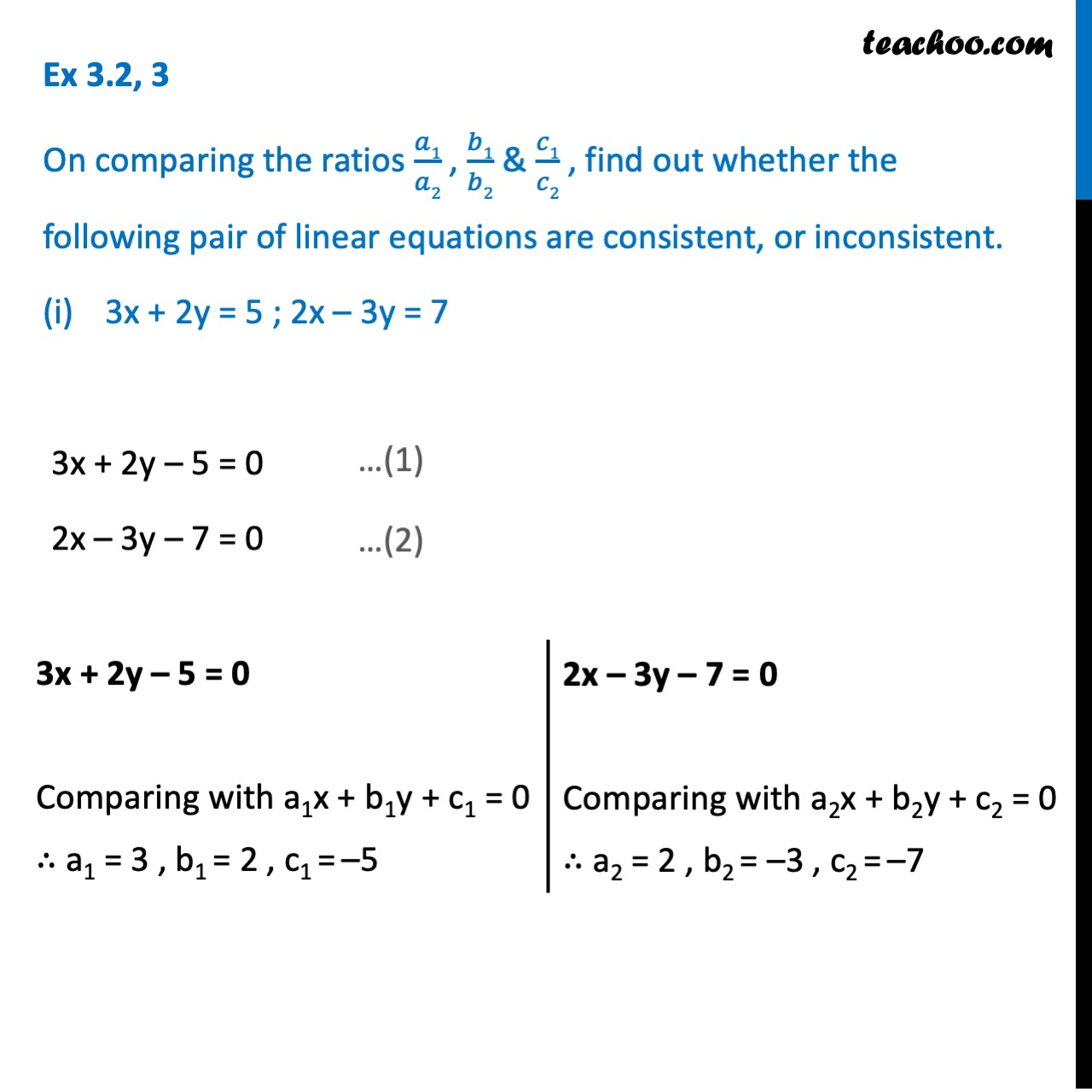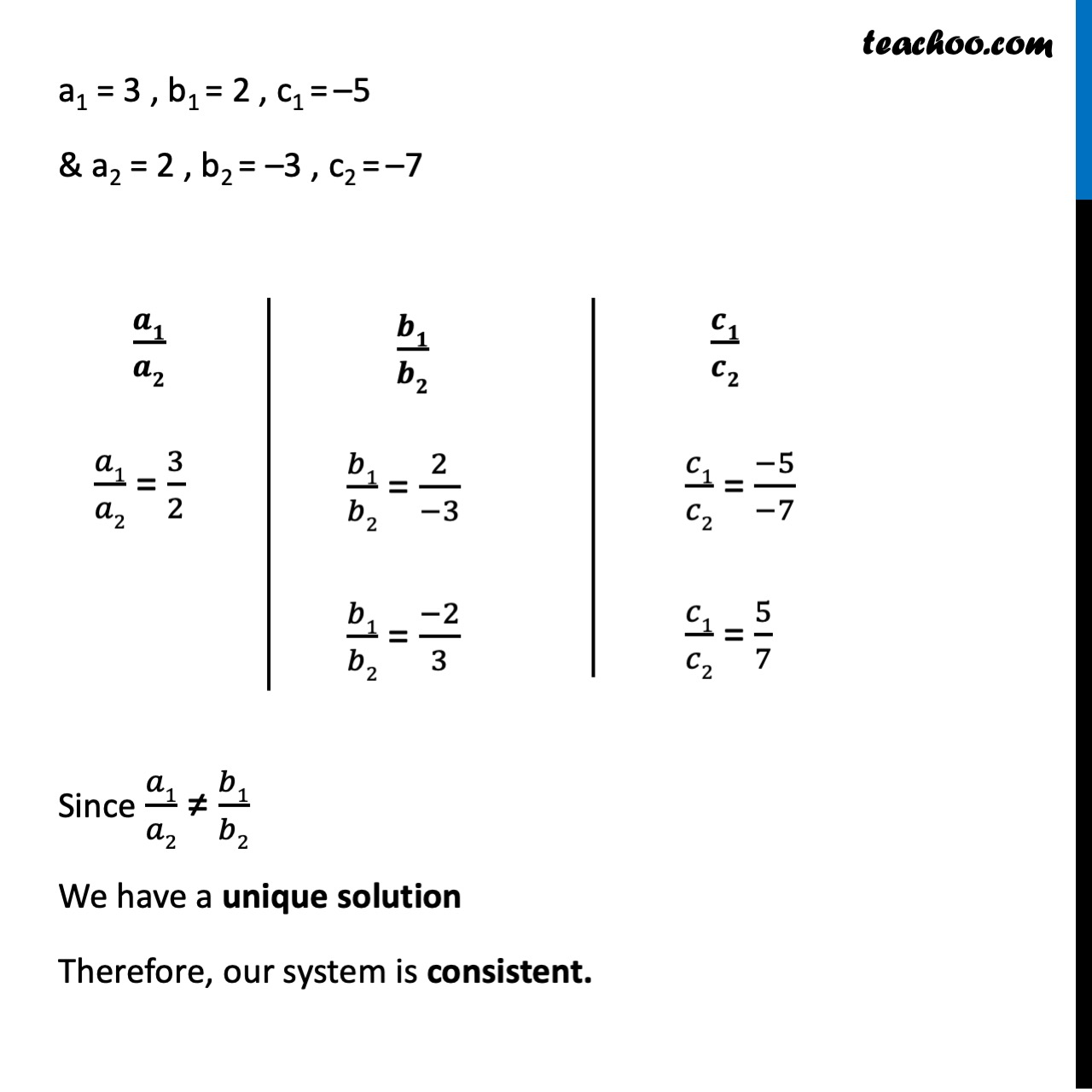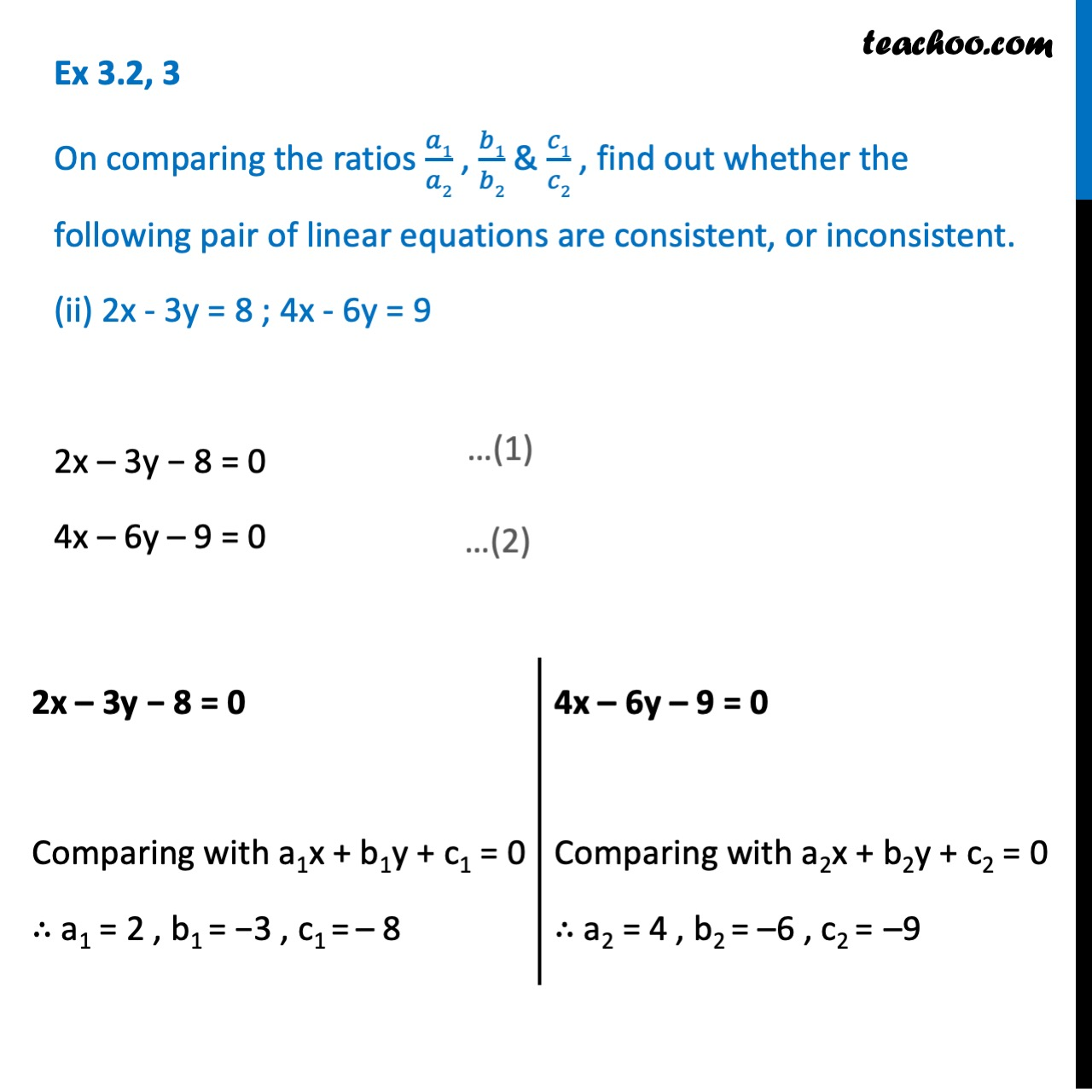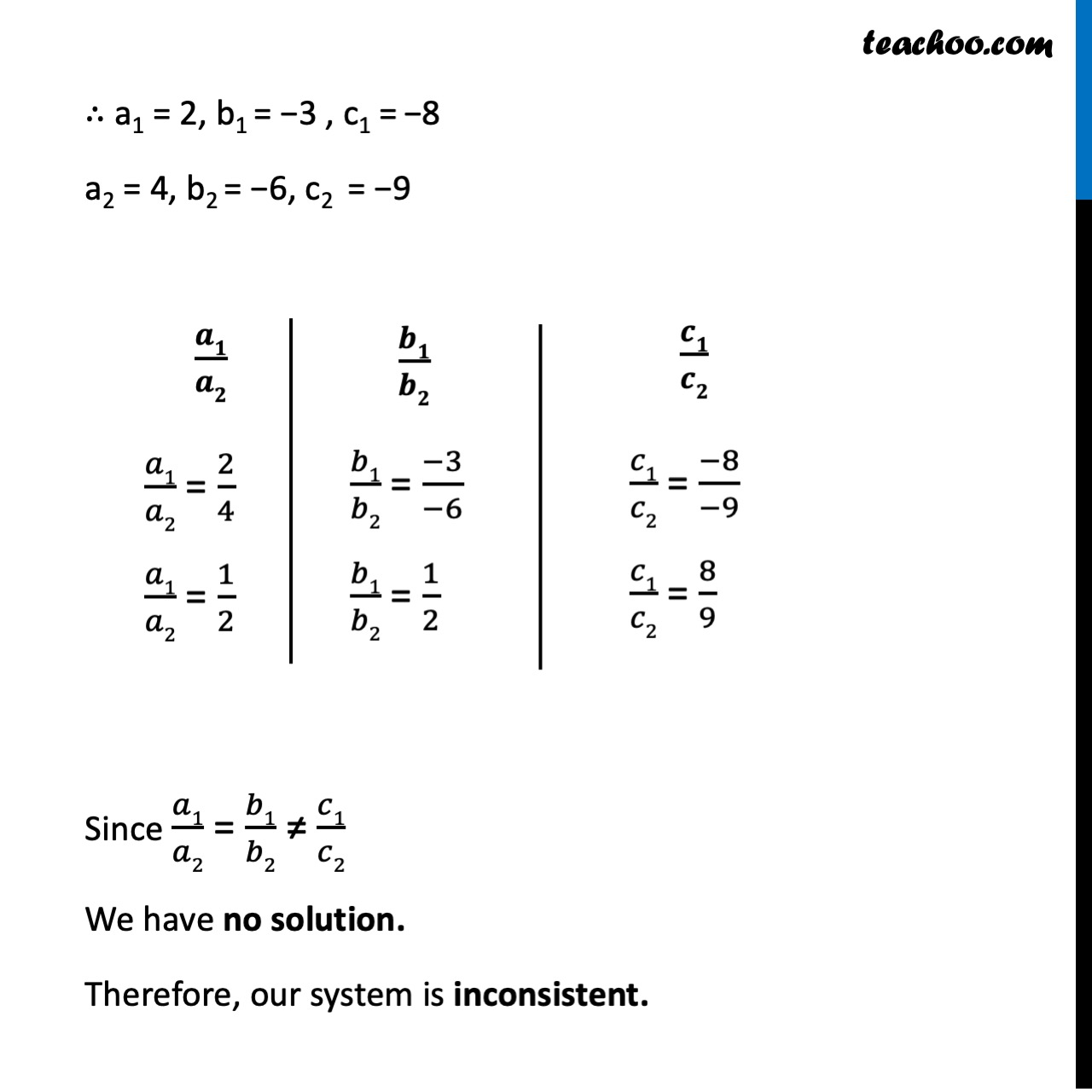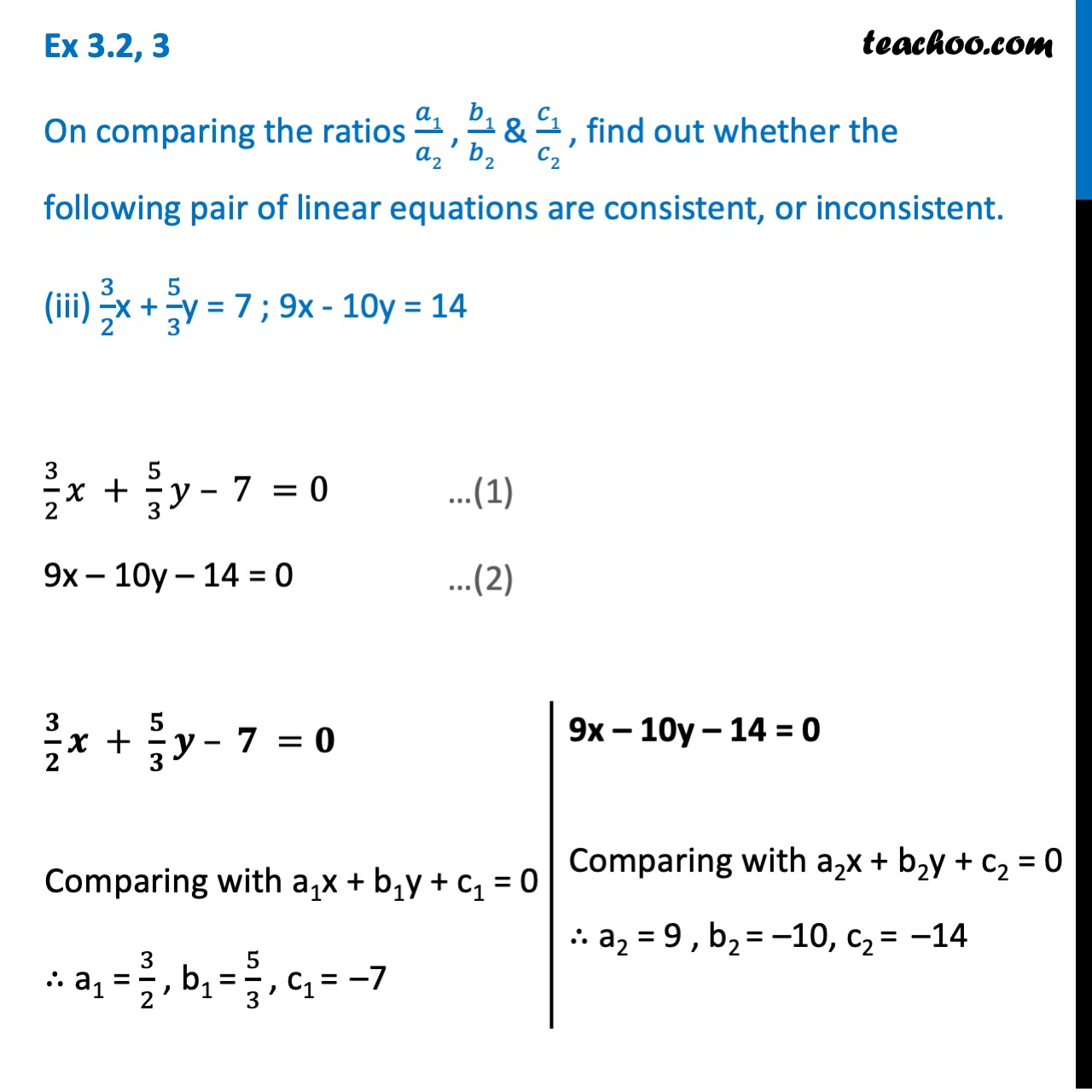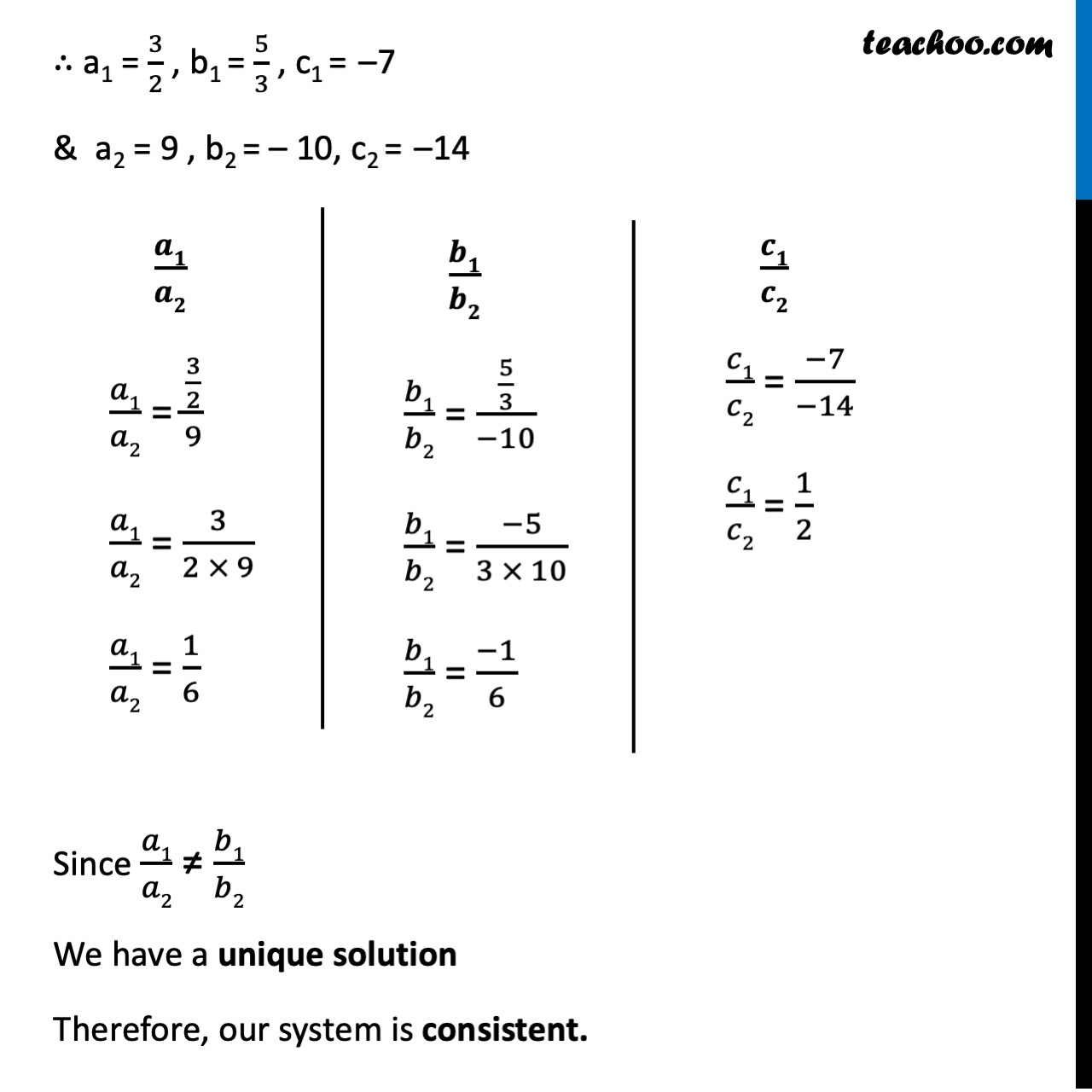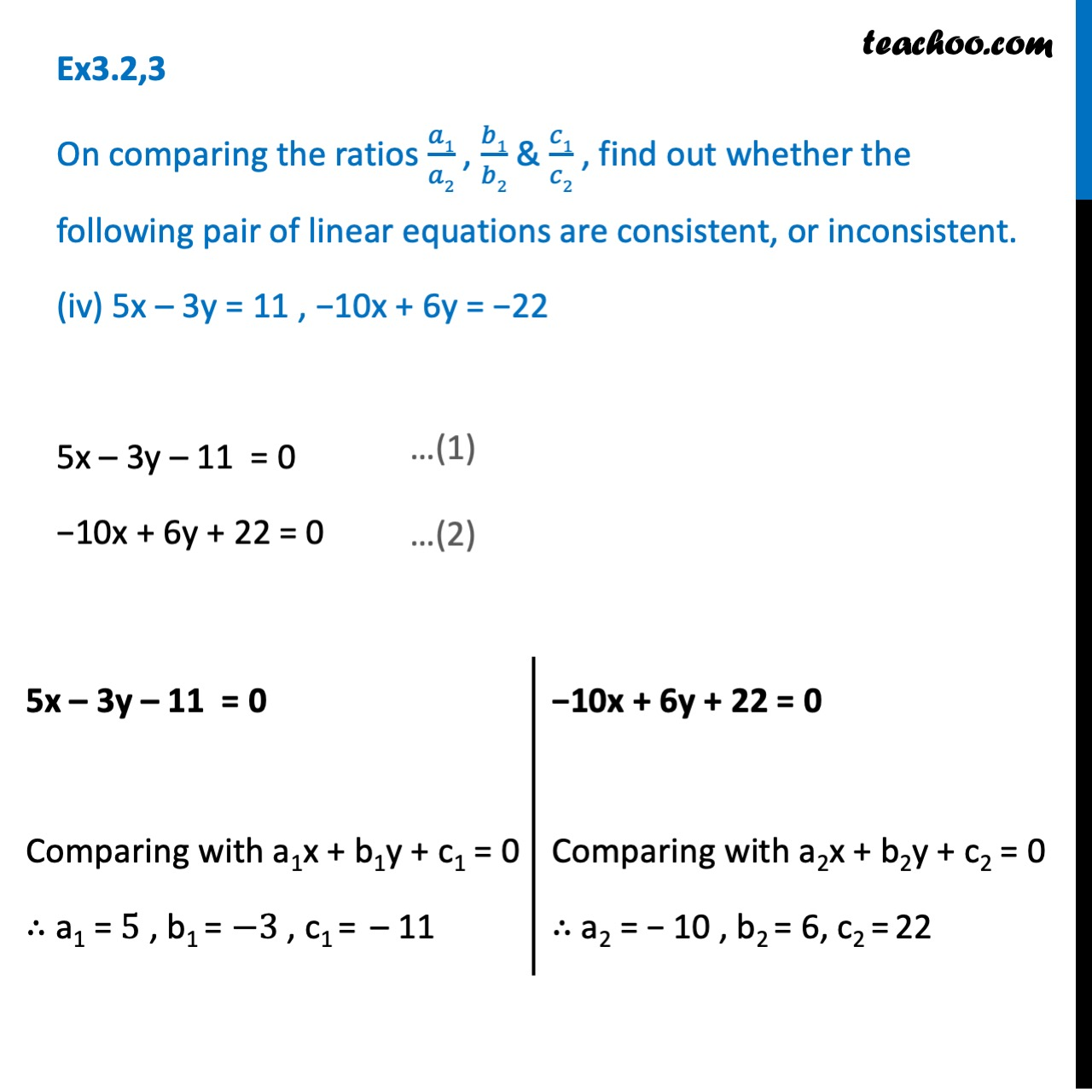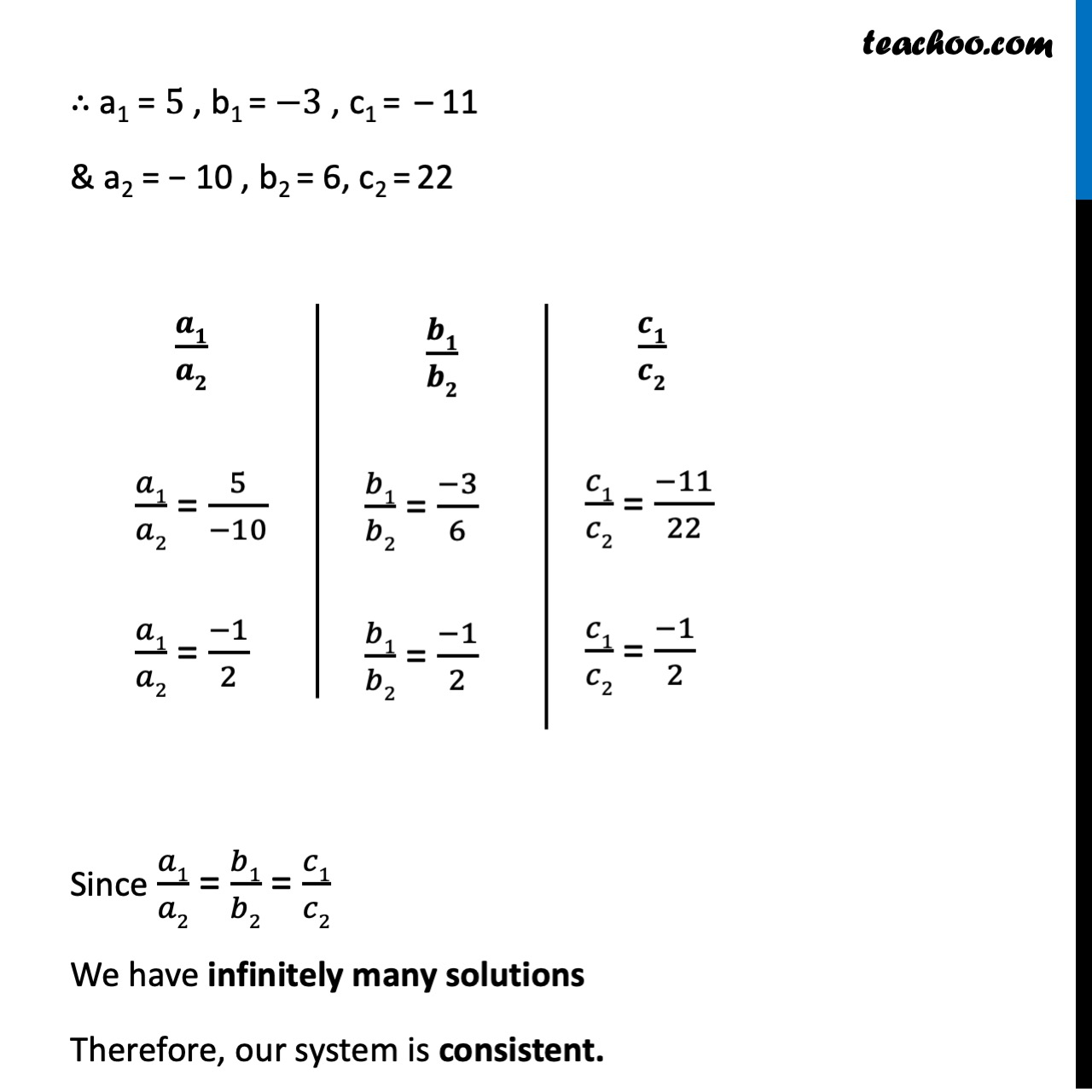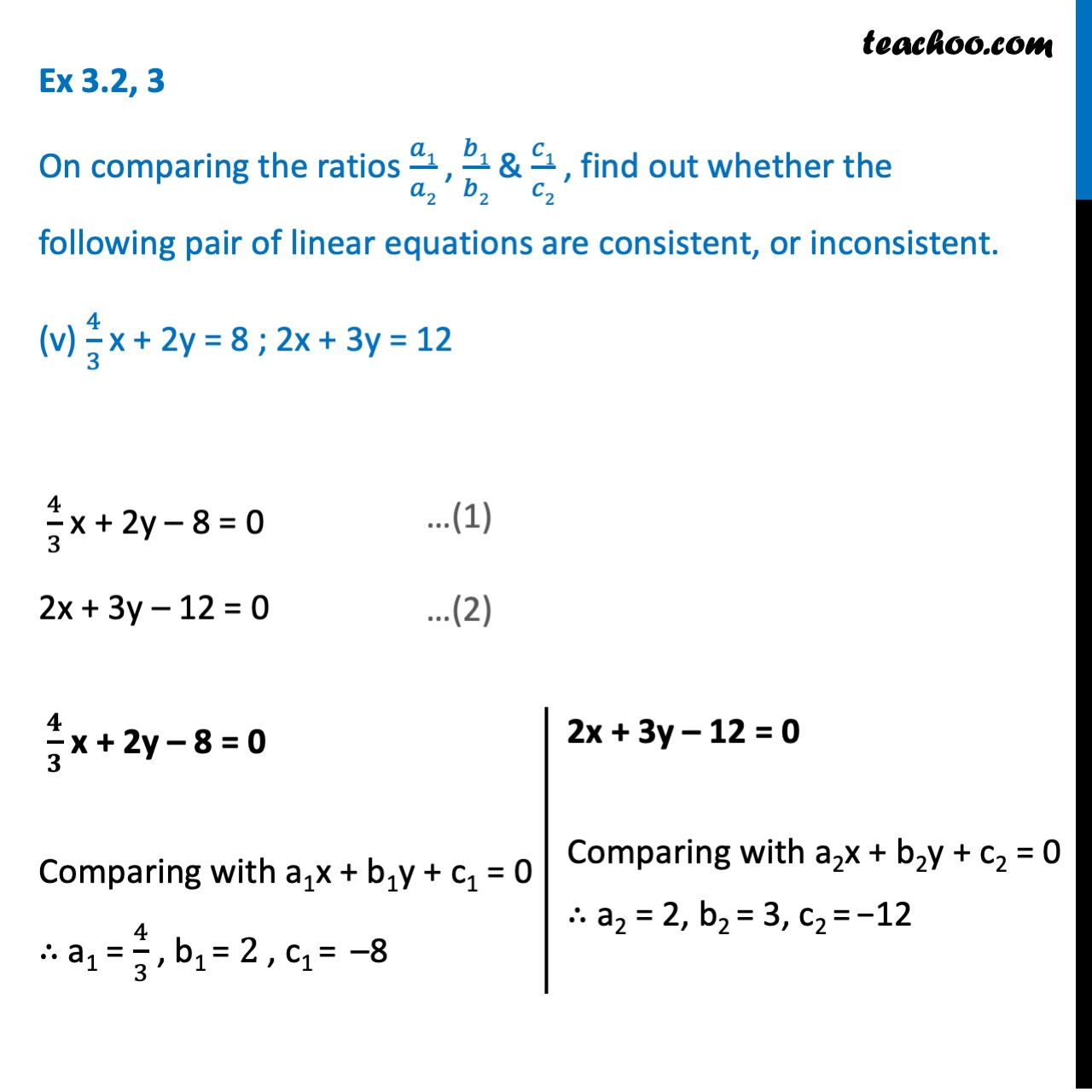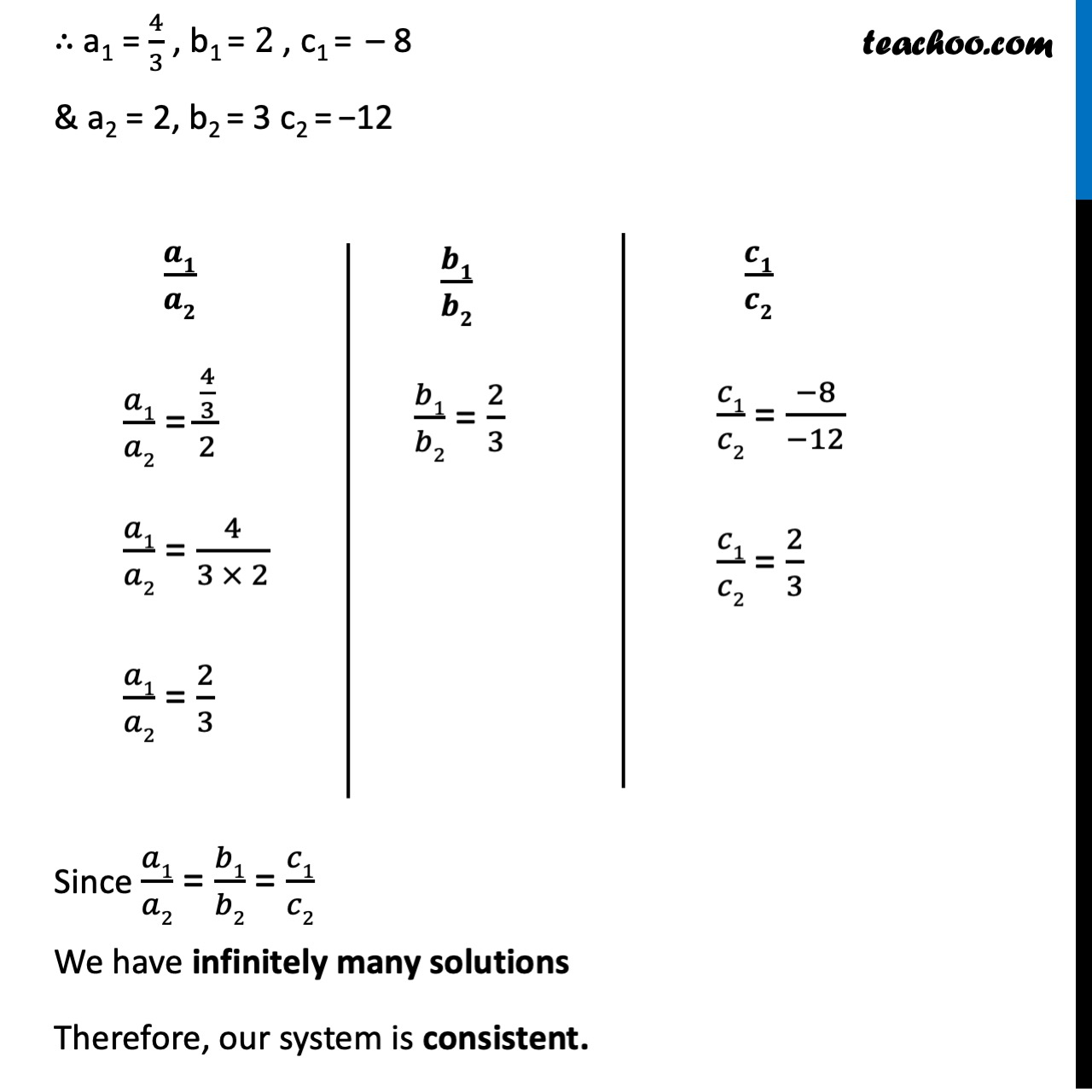1. Chapter 3 Class 10 Pair of Linear Equations in Two Variables
2. Serial order wise
3. Ex 3.2

Transcript

Ex 3.2, 3 On comparing the ratios 𝑎1/𝑎2 , 𝑏1/𝑏2 & 𝑐1/𝑐2 , find out whether the following pair of linear equations are consistent, or inconsistent. 3x+ 2y= 5 ; 2x– 3y= 7 3x + 2y – 5 = 0 2x – 3y – 7 = 0 3x + 2y – 5 = 0 Comparing with a1x + b1y + c1 = 0 ∴ a1 = 3 , b1 = 2 , c1 = –5 2x – 3y – 7 = 0 Comparing with a2x + b2y + c2 = 0 ∴ a2 = 2 , b2 = –3 , c2 = –7 a1 = 3 , b1 = 2 , c1 = –5 & a2 = 2 , b2 = –3 , c2 = –7 Since 𝑎1/𝑎2 ≠ 𝑏1/𝑏2 We have a unique solution Therefore, our system is consistent. 𝒂𝟏/𝒂𝟐 𝒃𝟏/𝒃𝟐 𝒄𝟏/𝒄𝟐 𝑎1/𝑎2 = 3/2 𝑏1/𝑏2 = 2/(−3) 𝑏1/𝑏2 = -2/3 𝑐1/𝑐2 = (−5)/(−7) 𝑐1/𝑐2 = 5/7 Ex 3.2, 3 On comparing the ratios 𝑎1/𝑎2 , 𝑏1/𝑏2 & 𝑐1/𝑐2 , find out whether the following pair of linear equations are consistent, or inconsistent. (ii) 2x -3y = 8 ; 4x - 6y = 9 2x – 3y − 8 = 0 4x – 6y – 9 = 0 2x – 3y − 8 = 0 Comparing with a1x + b1y + c1 = 0 ∴ a1 = 2 , b1 = − 3 , c1 = – 8 4x – 6y – 9 = 0 Comparing with a2x + b2y + c2 = 0 ∴ a2 = 4 , b2 = – 6 , c2 = – 9 ∴ a1 = 2, b1 = − 3 , c1 = − 8 a2 = 4, b2 = −6, c2 = − 9 Since 𝑎1/𝑎2 = 𝑏1/𝑏2 ≠ 𝑐1/𝑐2 We have no solution. Therefore, our system is inconsistent. 𝑎1/𝑎2 = 2/4 𝑎1/𝑎2 = 1/2 𝑏1/𝑏2 = (−3)/(−6) 𝑏1/𝑏2 = 1/2 𝑐1/𝑐2 = (−8)/(−9) 𝑐1/𝑐2 = 8/9 𝑐1/𝑐2 = (−8)/(−9) 𝑐1/𝑐2 = 8/9 Ex 3.2, 3 On comparing the ratios 𝑎1/𝑎2 , 𝑏1/𝑏2 & 𝑐1/𝑐2 , find out whether the following pair of linear equations are consistent, or inconsistent. (iii) 3/2x + 5/3y = 7 ; 9x - 10y = 14 3/2 𝑥 + 5/3 𝑦 – 7 =0 9x – 10y – 14 = 0 𝟑/𝟐 𝒙 + 𝟓/𝟑 𝒚 – 𝟕 =𝟎 Comparing with a1x + b1y + c1 = 0 ∴ a1 = 3/2 , b1 = 5/3 , c1 = – 7 9x – 10y – 14 = 0 Comparing with a2x + b2y + c2 = 0 ∴ a2 = 9 , b2 = – 10, c2 = – 14 𝑎1/𝑎2 = (3/2)/9 𝑎1/𝑎2 = 3/(2 × 9) 𝑎1/𝑎2 = 1/(2 𝑎×3) 𝑎1/𝑎2 = 1/6 𝑏1/𝑏2 = (5/3)/(−10) 𝑏1/𝑏2 = 5/(−3 ×10) 𝑏1/𝑏2 = 1/(−3 × 2) 𝑏1/𝑏2 = (−1)/6 𝑐1/𝑐2 = (−7)/(−14) 𝑐1/𝑐2 = 1/2 Since 𝑎1/𝑎2 ≠ 𝑏1/𝑏2 We have a unique solution Therefore, our system is consistent. Ex 3.2,3 On comparing the ratios 𝑎1/𝑎2 , 𝑏1/𝑏2 & 𝑐1/𝑐2 , find out whether the following pair of linear equations are consistent, or inconsistent. (iv) 5x – 3y = 11 , -10x + 6y = -22 5x – 3y – 11 = 0 −10x + 6y + 22 = 0 5x – 3y – 11 = 0 Comparing with a1x + b1y + c1 = 0 ∴ a1 = 5 , b1 = −3 , c1 = – 11 −10x + 6y + 22 = 0 Comparing with a2x + b2y + c2 = 0 ∴ a2 = − 10 , b2 = 6, c2 = 22 ∴ a1 = 5 , b1 = −3 , c1 = – 11 & a2 = − 10 , b2 = 6, c2 = 22 Since 𝑎1/𝑎2 = 𝑏1/𝑏2 = 𝑐1/𝑐2 We have infinitely many solutions Therefore, our system is consistent. 𝒂𝟏/𝒂𝟐 𝑎1/𝑎2 = 5/(−10) 𝑎1/𝑎2 = −1/2 𝑏1/𝑏2 = (−3)/6 𝑏1/𝑏2 = −1/2 𝑐1/𝑐2 = (−11)/22 𝑐1/𝑐2 = − 1/2 ∴ a1 = 5 , b1 = −3 , c1 = – 11 & a2 = − 10 , b2 = 6, c2 = 22 Since 𝑎1/𝑎2 = 𝑏1/𝑏2 = 𝑐1/𝑐2 We have infinitely many solutions Therefore, our system is consistent. Ex 3.2, 3 On comparing the ratios 𝑎1/𝑎2 , 𝑏1/𝑏2 & 𝑐1/𝑐2 , find out whether the following pair of linear equations are consistent, or inconsistent. (v) 4/3 x + 2y = 8 ; 2x + 3y = 12 4/3 x + 2y – 8 = 0 2x + 3y – 12 = 0 𝟒/𝟑 x + 2y – 8 = 0 Comparing with a1x + b1y + c1 = 0 ∴ a1 = 4/3 , b1 = 2 , c1 = – 8 2x + 3y – 12 = 0 Comparing with a2x + b2y + c2 = 0 ∴ a2 = 2, b2 = 3 c2 = −12 𝑎1/𝑎2 = (4/3)/2 𝑎1/𝑎2 = 4/(3 × 2) 𝑎1/𝑎2 = 2/(3 × 1) 𝑎1/𝑎2 = 2/3 𝑏1/𝑏2 = 2/3 𝑐1/𝑐2 = (−8)/(−12) 𝑐1/𝑐2 = 2/3 Since 𝑎1/𝑎2 = 𝑏1/𝑏2 = 𝑐1/𝑐2 We have infinite solutions. Therefore, our system is consistent.

Ex 3.2